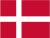### Dish Cloth 45

Cast on  61 stitches and knit 6 rows and then pattern.
Remember to start all uneven rows with 1 k and end all even rows with 1 p, when you knit the pattern. Knit the pattern 2 times and then moss stitch *1 k,1 p*, until the cloth is 16 cm.Then you knit the pattern again 2 times. Knit 6 rows and bind off.

Pattern::
1.row: knit
2.row: 2 p, 3 k, 3 p, 3 k,1 p
3.row: 1 p, 1 k, 3 p, 1 k, 3 p, 1 k, 1 p, 1 k
4.row: 1 p, 2 k, 1 p, 2 k, 1 p, 2 k, 1 p, 2 k
5.row: 3 p, 1 k, 1 p, 1 k, 1 p, 1 k, 3 p, 1 k
6.row: as row 2
7.row: knit
8.row: as row 2
9.row: as row 5
10.row: as row 4
11.row: as row 3
12.row: as row 2

 X X X X X X 12 X X X X X X X X 11 X X X X X X X X 10 X X X X X X X X 9 X X X X X X 8 7 X X X X X X 6 X X X X X X X X 5 X X X X X X X X 4 X X X X X X X X 3 X X X X X X 2 1
You have to remember that all uneven rows start in the right side of the diagram and X=purl and ▢= knit. All equal rows start in the left side of the diagram and X=knit and ▢=purl.Danish